8 篇文章 0 订阅

# 多元线性回归分析

## 多元线性回归分析

• 相关性
通过回归分析，研究相关关。相关性不等于因果性
• X
X是用来解释Y的相关变量，所以X被称为自变量。
• Y
Y常常是我们需要研究的那个核心变量，称为因变量。

### 回归分析的分类

0-1回归logistic回归二值变量是否违约、是否得病

### 一元线性回归

• 回归模型
只涉及一个自变量的回归称为一元回归，描述两个具有线性关系的变量之间关系的方程称为回归模型，一元线性回归模型可表示为：
y i = β 0 + β 1 x i + μ i y_i=\beta_0+\beta_1x_i+\mu_i

• y与x之间具有线性关系；
• x是非随机的，在重复抽样中，x的取值是固定的；
以上2个假定表明，对于任何一个给定的x的值，y的取值都对应着一个分布，代表一条直线。但由于单个y是从y的分布中抽出来的，可能不在这条直线上，因此，必须包含一个误差项。
• 误差项是一个期望值为0的随机变量，因此，对于一个给定的x值，y的期望 E ( y ) = β 0 + β 2 x E(y)=\beta_0+\beta_2x 值。
• 对于所有的x， μ \mu 的方差 σ \sigma 都相同，这意味着对于一个给定的x值，y的方差都等于 σ 2 \sigma^2
• 误差项是一个服从正态分布的随机变量，且独立。一个特定的x值所对应的与其他x值对应的不相关。对于任何一个给定的x值，y都服从期望值为 β 0 + β 1 x \beta_0+\beta_1x 方差为 σ 2 \sigma^2 的正态分布，不同的x值，y的期望值不同，但方差相同

y i = β 0 + β 1 x i + μ i y_i=\beta_0+\beta_1x_i+\mu_i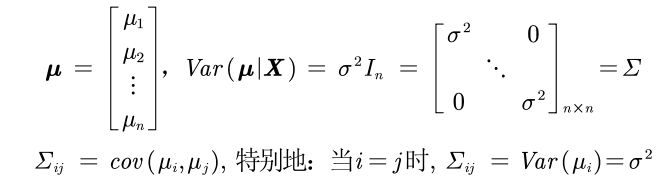• 回归方程
描述y的期望值如何依赖自变量x的方程称为回归方程，一元线性回归方程（误差项的期望值为0）的形式为：
E ( y ) = β 0 + β 2 x E(y)=β_0+β_2x
• 估计的回归方程
总体回归参数 β 0 β_0 β 1 β_1 是未知的，需要用样本数据去估计。一元线性回归的估计的回归方程形式为：

y ^ = β ^ 0 + β ^ 1 x \hat y=\hat\beta_0+\hat\beta_1x

• 对于“线性”的理解
不要求严格的线性，只要有线性的形式即可。
如下，都属于“线性”。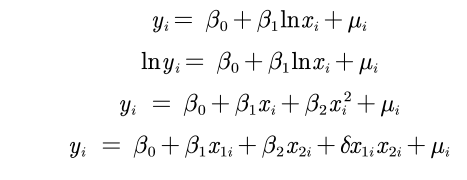## 内生性的探究

### 蒙特卡罗模拟

%% 蒙特卡洛模拟：内生性会造成回归系数的巨大误差
times = 300;  % 蒙特卡洛的次数
R = zeros(times,1);  % 用来储存扰动项u和x1的相关系数
K = zeros(times,1);  % 用来储存遗漏了x2之后，只用y对x1回归得到的回归系数
for i = 1: times
n = 30;  % 样本数据量为n
x1 = -10+rand(n,1)*20;   % x1在-10和10上均匀分布，大小为30*1
u1 = normrnd(0,5,n,1) - rand(n,1);  % 随机生成一组随机数
x2 = 0.3*x1 + u1;   % x2与x1的相关性不确定， 因为我们设定了x2要加上u1这个随机数
% 这里的系数0.3我随便给的，没特殊的意义，你也可以改成其他的测试。
u = normrnd(0,1,n,1);  % 扰动项u服从标准正态分布
y = 0.5 + 2 * x1 + 5 * x2 + u ;  % 构造y
k = (n*sum(x1.*y)-sum(x1)*sum(y))/(n*sum(x1.*x1)-sum(x1)*sum(x1)); % y = k*x1+b 回归估计出来的k
K(i) = k;
u = 5 * x2 + u;  % 因为我们回归中忽略了5*x2，所以扰动项要加上5*x2
r = corrcoef(x1,u);  % 2*2的相关系数矩阵
R(i) = r(2,1);
end
plot(R,K,'*')
xlabel("x_1和u'的相关系数")

ylabel("k的估计值")## 回归系数的解释

### 一元线性回归

y = a + b x + μ y=a+bx+\mu
β 0 ^ \hat{\beta_0} : 一般不考虑（所有变量都是0才有意义）

β m ^ ( m = 1 , 2... k ) \hat{\beta_m}(m=1,2...k) ：在控制了其他变量的情况下， x m i x_{mi} 每增加一个单位，对 y i y_i 造成的变化。

### 半对数模型1

y = a + b l n x + μ y=a+blnx+\mu

• 与市场价值相关的，例如，价格、销售额、工资等都可以取对数；
• 以年度量的变量，如受教育年限、工作经历等通常不取对数；
• 比例变量，如失业率、参与率等，两者均可；
• 变量取值必须是非负数，如果包含0，则可以对y取对数ln(1+y);

• 减弱数据的异方差性
• 如果变量本身不符合正态分布，取了对数后可能渐近服从正态分布
• 模型形式的需要，让模型具有经济学意义
x每增加1%，y平均变化b/100个单位。

### 半对数模型2

l n y = a + b x + μ lny=a+bx+\mu
x每增加1个单位，y平均变化(100b)%。

### 双对数模型

l n y = a + b l r n x + μ lny=a+blrnx+\mu
x每增加1%，y平均变化b%

## 虚拟变量

• 如果自变量中有定性变量，例如性别、地域等，在回归中转化为0-1表示
• 为了避免完全多重共线性的影响，引入虚拟变量的个数一般是分类数减1。

## 数据的描述性统计

### stata 实现

• 定量数据summarize 变量1 变量2 ... 变量n
• 定性数据tabulate 变量名,gen(A)
返回对应的这个变量的频率分布表，并生成对应的虚拟变量(以A开头)。gen以后的不写也可以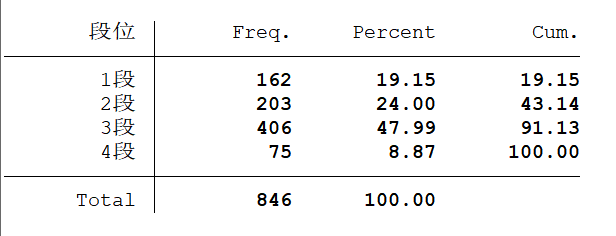• Freq 频数
• Percent 频率
• Cum 累积频率

### Excel实现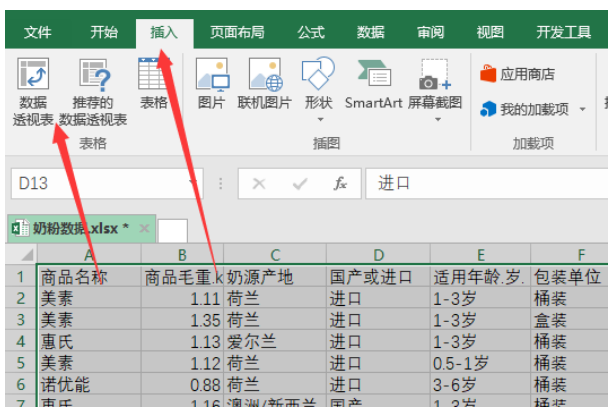## 回归实现

STATA语句 ： regress y x1 x2 … xk

• 默认使用的OLS：普通最小二乘估计法）
• 如果假如虚拟变量 STATA会自动检测数据的完全多重共线性
• 不带虚拟变量的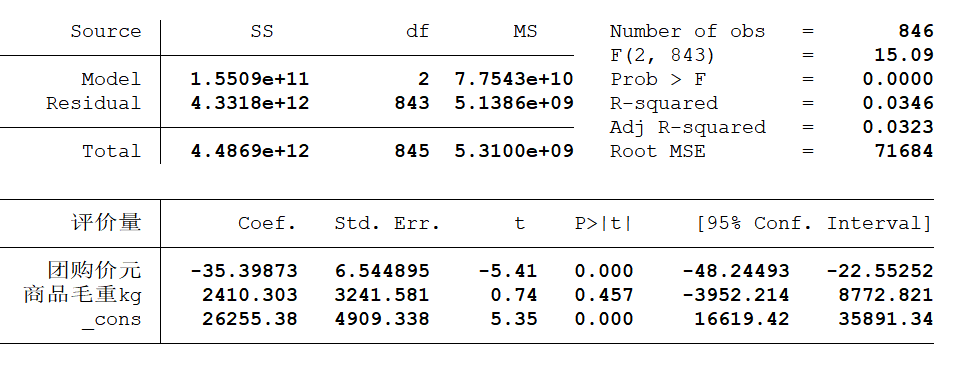• Model + SS :SSR回归平方和
• Residual +SS : SSE误差平方和
• Total + SS ：SST=SSR+SSE
• df : 自由度
• MS ：ss/df
• F&&Prob>F : 联合显著性检验。 H 0 : β 1 = β 2 = . . . β k = 0 H_0 : \beta_1=\beta_2=...\beta_k=0
• R 2 a n d a d j R 2 R^2 and adj R^2 :一般使用调整后的
• _cons : 常数项
• H 0 : β 1 = β 2 = . . . β k = 0 H_0 : \beta_1=\beta_2=...\beta_k=0
• 带虚拟变量的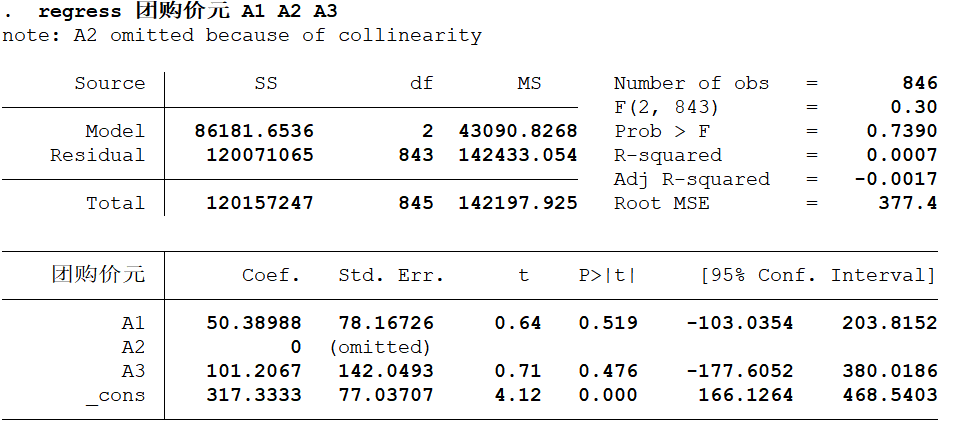避免多重共线性，把一个固定（为0）， 其余的数值是与它作比较。

• 回归分为解释型回归和预测型回归。
预测型回归一般才会更看重 R 2 R^2 。解释型回归更多的关注模型整体显著性以及自变量的统计显著性和经济意义显著性即可。
• 可以对模型进行调整，例如对数据取对数或者平方后再进行回归。
• 数据中可能有存在异常值或者数据的分布极度不均匀。
• 我们引入的自变量越多，拟合优度会变大。但我们倾向于使用调整后的拟合优度，如果新引入的自变量对SSE的减少程度特别少，那么调整后的拟合优度反而会减小。

## 标准化回归

### Stata标准化回归命令

regress y x1 x2 … xk,beta
bata可简写为b

• 常数项没有标准化回归系数
常数的均值是其本身，经过标准化后变成了0。
-除了多了标准化回归系数 ,和之前的回归结果完全相同。
对数据进行标准化处理不会影响回归系数的标准误，也不会影响显著性。

## 异方差

• 危害
当扰动项存在异方差时 ：
• OLS估计出来的回归系数是无偏、一致的。
• 假设检验无法使用（构造的统计量失效了）。
• OLS估计量不再是最优线性无偏估计量（BLUE）。
• 检验(by stata)
\\在回归结束后运行命令：
rvfplot \\(画残差与拟合值的散点图）
rvpplot x \\(画残差与自变量x的散点图)
estat hettest ,rhs iid\\异方差bp检验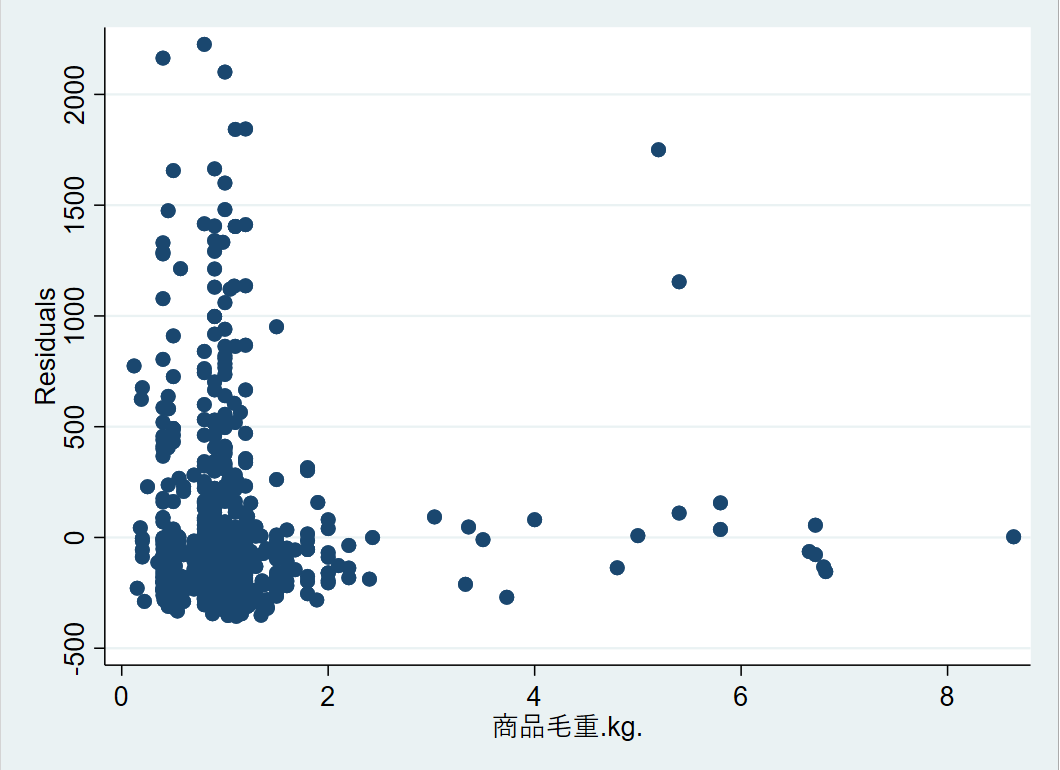• BP检验
• 原假设 : 扰动项不存在异方差
• 备选假设 ：扰动项存在异方差
• 解决方案
1. 使用OLS + 稳健的标准误
如果发现存在异方差，一 种处理方法是，仍然进行OLS 回归，但使用稳健标准误。只要样本容量较大，即使在异方差的情况下，若使用稳健标准误，则所 有参数估计、假设检验均可照常进行。
regress y x1 x2 … xk,robust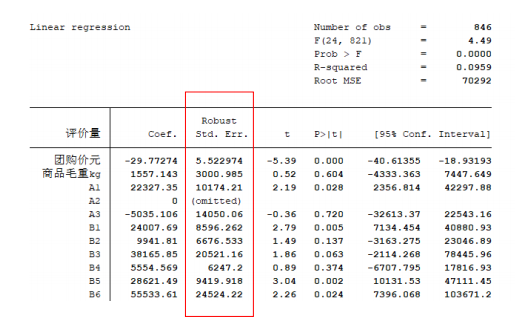Stock and Watson (2011)推荐，在大多数情况下应该使用“OLS + 稳健标准误”。
2. 广义最小二乘估计法GLS（有缺陷）

## 多重共线性

• 检验多重共线性
VIF，VarianceInflation Factor，方差膨胀因子。VIF指的是解释变量之间存在多重共线性时的方差与不存在多重共线性时的方差之比，可以反映多重共线性导致的方差的增加程度
V I F m VIF_m 越大，说明第 m m 个变量和其他变量的相关性越大，回归模型的 V I F = m a x { V I F 1 , V I F 2 , . . . , V I F K } VIF=max\{VIF_1,VIF_2,...,VIF_K\}
一般 V I F > 10 VIF>10 认为回归方程存在严重的多重共线性。
\\Stata计算各自变量VIF的命令（在回归结束后使用）：
estat vif

• 如果不关心具体的回归系数，而只关心整个方程预测被解释变量的能力，则通常可以 不必理会多重共线性（假设你的整个方程是显著的）。这是因为，多重共线性的主要后果是使得对单个变量的贡献估计不准，但所有变量的整体效应仍可以较准确地估计。
• 如果关心具体的回归系数，但多重共线性并不影响所关心变量的显著性，那么也可以不必理会。即使在有方差膨胀的情况下，这些系数依然显著;如果没有多重共线性，则只会更加显著。
• 如果多重共线性影响到所关心变量的显著性，则需要增大样本容量，剔除导致严重共线性的变量（不要轻易删除，因为可能会有内生性的影响），或对模型设定进行修改。
• 逐步回归分析
• 向前逐步回归Forward selection：将自变量逐个引入模型，每引入一个自变量后都要进行检验，显著时才加入回归模型。
(缺点：随着以后其他自变量的引入，原来显著的自变量也可能又变为不显著了，但是，并没有将其及时从回归方程中剔除掉。)
• 向后逐步回归Backward elimination：与向前逐步回归相反，先将所有变量均放入模型，之后尝试将其中一个自变量从模型中剔除，看整个模型解释因变量的变异是否有显著变化，之后将最没有解释力的那个自变量剔除；此过程不断迭代，直到没有自变量符合剔除的条件。
（缺点：一开始把全部变量都引入回归方程，这样计算量比较大。若对一些不重要的变量，一开始就不引入，这样就可以减少一些计算。当然这个缺点随着现在计算机的能力的提升，已经变得不算问题了）
• Stata实现逐步回归法
\\向前逐步回归Forward selection：
stepwise regress y x1 x2 … xk, pe(#1)

\\向后逐步回归Backward elimination：
stepwise regress y x1 x2 … xk, pr(#2)


• pe(#1) specifies the significance level for addition to the model; terms with p<#1 are eligible for addition（显著才加入模型中）.
• pr(#2) specifies the significance level for removal from the model; terms with p>= #2 are eligible for removal（不显著就剔除出模型）
• 如果你筛选后的变量仍很多，可以减小#1或者#2;如果筛选后的变量太少了，可以增加#1或者#2。
• x 1 x 2 … x k x_1 x_2 … x_k 之间不能有完全多重共线性(和regress不同哦)
• 可以在后面再加参数b和r，即标准化回归系数或稳健标准误
-向前逐步回归和向后逐步回归的结果可能不同。
• 不要轻易使用逐步回归分析，因为剔除了自变量后很有可能会产生新的问题，例如内生性问题。
04-04
08-081万+09-11
07-031万+
09-181万+
11-14
02-17
04-03568
09-242万+
11-27112
02-232334
04-015万+
09-18点击重新获取扫码支付余额充值